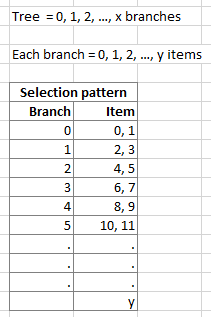# Select items from the tree

This is a question about selecting items from the tree in a specific way.

I have a tree that has x number of branches. From branch 0 to n, each branch has y number of items (the number of items remains same in each branch). Now I want to select two items from one branch and the next two items from the next branch until y items are selected (have a look at the image for example). Now x and y are variables where x>y, x<y or x=y, anything is possible. It would be great if someone can help me to solve this puzzle in grasshopper. To start with, one can choose x=17 and y=24. But keeping them variable would be the best.You can use Sublist (0-1) to get first two items from each branch, then flatten (or shift path) the resulted tree and use sublist again with 0 to Y domain

this should do exactly what you are looking for:

select items from tree_Re.gh (17.1 KB)

 question: it is unclear to me why you want the number of branches from which data is collected (x) to be an explicit input, instead of being gathered directly from the data-tree itself like the following:

if you want to collect items just from the (let’s say) first 10 branches, knowing you’ll get 2 items from each branch, all you have to do is set y=20